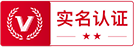##用户名 Email 自动登录 找回密码 密码 立即注册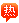# ∑是什么意思？

2020-7-17 16:02| 发布者: admin| 查看: 32| 评论: 0

### cosimayuwang的回答

∑的用法：

∑ i 这样表达也可以，表示对i求和，i是变数。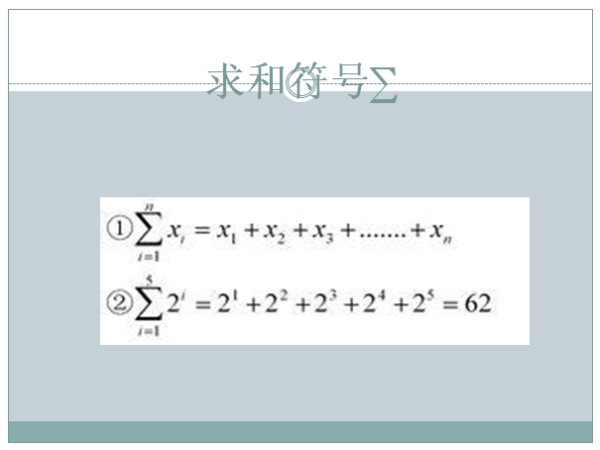1+2+3+···n=n(n+1)/2。

### Solely时潋的回答

Σ是希腊字母表中24个字母中的第18个大写字母，读音sigma（中音谐 西格玛），小写是σ。

=1+2+3+......+(n-1)+n=n(n+1)/2∑ (求和符号）

### lkxlly的回答

∑是求和符号
4
∑ 0.5i=0.5×1＋0.5×2＋0.5×3＋0.5×4 =5
i=1

### 132231097的回答

∑是数列求和的简记号，它后面的k^2是通项公式，下面的k=1是初始项开始的项数，顶上的n是末项的项数。
n
∑k^2=1^2+2^2+……+n^2……（1）
k=1
n
∑(2k+1)=3+5+……+(2n+1)……（2）
k=1

n
∑(k+1)^2=2^2+3^2+……+（n+1）^2
k=1

n
∑C(n,k)a^(n-k)b^k.
k=0

### Foolicial的回答

∑是希腊大写字母，读作sigma（西格玛）

n
∑ i^2=1^2+2^2+……+n^2
i=1

∑（a+b）^2=(a+b)^2+(b+c)^2+(c+a)^2
cyc

### 最新评论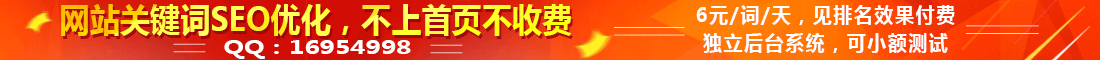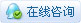|广告服务|网站出售|投稿要求|禁言标准|版权说明|免责声明|手机版|小黑屋|推扬网 ( 粤ICP备18134897号 )|网站地图

GMT+8, 2021-1-25 06:00 , Processed in 0.560663 second(s), 29 queries .